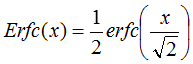# Error Function erf(x) & Complementary Error Function erfc(x)

Note: The error function and complementary function used in communications are not exactly the same as the ones typically used in statistics. The relationship between the two is given at the bottom of the page.

"In mathematics, the error function (also called the Gauss error function) is a special function (non-elementary) of sigmoid shape which occurs in probability, statistics, materials science, and partial differential equations." - Wikipedia

The Gaussian probability density function with mean = 0 and variance =1 isThe error function erf(x) is defined as:Note that erf(0) = 0.5, and that erf(∞)=1.

The complementary error function erfc(x) is defined as:The following graph illustrates the region of the normal curve that is being integrated.For large values of x (>3), the complementary error function can be approximated by:The error in the approximation is about -2% for x=3, and -1% for x=4, and gets progressively better with larger values of x.

## Approximations

RF Cafe visitor Ilya L. provided an approximation for the error function and complementary error function that was published by Sergei Winitzki titled, "A handy approximation for the error function and its inverse," February 6, 2008 (Google Drive file - slow to load).

Here are the main results:

Error function approximation:

,whereComplementary error function:NOTE: I used to have an alternative approximation formula for the complementary error function for large values of x, but decided to remove it since the source for it is not generally available to the public. It can be found as equation #13, on page 641, of IEEE Transactions on Communications volume COM-27, No. 3, dated March 1979. A subscription to the IEEE service is required to access the article.About RF CafeCopyright: 1996 - 2024Webmaster:    Kirt Blattenberger,    BSEE - KB3UON RF Cafe began life in 1996 as "RF Tools" in an AOL screen name web space totaling 2 MB. Its primary purpose was to provide me with ready access to commonly needed formulas and reference material while performing my work as an RF system and circuit design engineer. The Internet was still largely an unknown entity at the time and not much was available in the form of WYSIWYG ... All trademarks, copyrights, patents, and other rights of ownership to images and text used on the RF Cafe website are hereby acknowledged. My Hobby Website:  AirplanesAndRockets.com